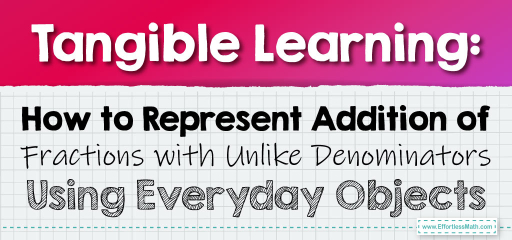# Tangible Learning: How to Represent Addition of Fractions with Unlike Denominators Using Everyday Objects

While adding fractions with different denominators can be a challenging concept for many, using tangible objects can make the process more intuitive and relatable.Everyday objects provide a hands-on approach to visualize and understand the addition of fractions. In this blog post, we’ll explore how to represent the addition of fractions with unlike denominators using objects you can find around you.

## Step-by-step Guide:

1. Understanding the Concept:

When adding fractions, especially those with different denominators, it’s essential to find a common ground. This “common ground” is known as the common denominator.

Select objects that can be easily divided into parts, such as pizza slices, chocolate bars, or sets of blocks. Ensure you have enough objects to represent the denominators of the fractions you’re working with.

3. Dividing the Objects:

Divide your chosen objects based on the denominators of the fractions. For instance, if you’re working with $$\frac{1}{4}$$ and $$\frac{3}{8}$$, divide a chocolate bar into 4 equal parts and another one into 8 equal parts.

4. Finding a Common Denominator Using Objects:

To add the fractions, they need to have the same denominator. Using the objects, find a size that both sets of objects can fit into. In our example, 8 is the common denominator since both 4 and 8 can fit into it.

5. Representing the Fractions with Objects:

Using the objects, represent each fraction. For $$\frac{1}{4}$$, take one part out of the four. For $$\frac{3}{8}$$, take three parts out of the eight.

6. Adding the Fractions Using Objects:

Combine the parts from both fractions. Count the total number of parts you have and represent it over the common denominator. In our example, you’ll have a total of 5 parts out of 8, representing $$\frac{5}{8}$$.

### Example 1:

Add $$\frac{2}{3}$$ and $$\frac{1}{6}$$ using blocks.

Solution:

If you divide a set of blocks into 3 parts and another set into 6 parts, the common denominator is 6. Representing the fractions, you’ll have 4 blocks for $$\frac{2}{3}$$ and 1 block for $$\frac{1}{6}$$. Combining them, you get 5 blocks out of 6, or $$\frac{5}{6}$$.

The Absolute Best Book for 5th Grade Students

### Example 2:

Add $$\frac{3}{5}$$ and $$\frac{1}{10}$$ using pizza slices.

Solution:

Dividing a pizza into 5 slices and another into 10 slices, the common denominator is 10. Representing the fractions, you’ll have 6 slices for $$\frac{3}{5}$$ and 1 slice for $$\frac{1}{10}$$. Combining them, you get 7 slices out of 10, or $$\frac{7}{10}$$.

### Practice Questions:

1. Add $$\frac{1}{4}$$ and $$\frac{2}{8}$$ using chocolate bars.

2. Add $$\frac{3}{7}$$ and $$\frac{2}{14}$$ using sets of blocks.

3. Add $$\frac{2}{9}$$ and $$\frac{1}{3}$$ using pizza slices.

A Perfect Book for Grade 5 Math Word Problems!

1. $$\frac{1}{2}$$

2. $$\frac{4}{7}$$

3. $$\frac{5}{9}$$

The Best Math Books for Elementary Students

### What people say about "Tangible Learning: How to Represent Addition of Fractions with Unlike Denominators Using Everyday Objects - Effortless Math: We Help Students Learn to LOVE Mathematics"?

No one replied yet.

X
51% OFF

Limited time only!

Save Over 51%

SAVE $15 It was$29.99 now it is \$14.99